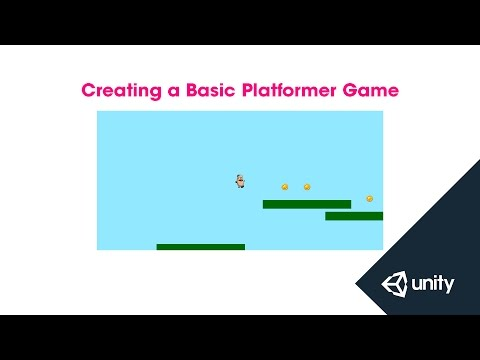# Creating a Basic Platformer Game

#### -

In this live training session we're going to prototype a simple platformer game. We'll cover player movement, create a simple level and add collectible objects.## Creating a Basic Platformer Game

Anfänger 2D Game Creation

### Code snippet

``````using UnityEngine;
using System.Collections;

public class SimplePlatformController : MonoBehaviour {

[HideInInspector] public bool facingRight = true;
[HideInInspector] public bool jump = false;
public float moveForce = 365f;
public float maxSpeed = 5f;
public float jumpForce = 1000f;
public Transform groundCheck;

private bool grounded = false;
private Animator anim;
private Rigidbody2D rb2d;

// Use this for initialization
void Awake ()
{
anim = GetComponent<Animator>();
rb2d = GetComponent<Rigidbody2D>();
}

// Update is called once per frame
void Update ()
{
grounded = Physics2D.Linecast(transform.position, groundCheck.position, 1 << LayerMask.NameToLayer("Ground"));

if (Input.GetButtonDown("Jump") && grounded)
{
jump = true;
}
}

void FixedUpdate()
{
float h = Input.GetAxis("Horizontal");

anim.SetFloat("Speed", Mathf.Abs(h));

if (h * rb2d.velocity.x < maxSpeed)

if (Mathf.Abs (rb2d.velocity.x) > maxSpeed)
rb2d.velocity = new Vector2(Mathf.Sign (rb2d.velocity.x) * maxSpeed, rb2d.velocity.y);

if (h > 0 && !facingRight)
Flip ();
else if (h < 0 && facingRight)
Flip ();

if (jump)
{
anim.SetTrigger("Jump");
jump = false;
}
}

void Flip()
{
facingRight = !facingRight;
Vector3 theScale = transform.localScale;
theScale.x *= -1;
transform.localScale = theScale;
}
}``````

### Code snippet

``````using UnityEngine;
using System.Collections;

public class SpawnPlatforms : MonoBehaviour {

public int maxPlatforms = 20;
public GameObject platform;
public float horizontalMin = 7.5f;
public float horizontalMax = 14f;
public float verticalMin = -6f;
public float verticalMax = 6;

private Vector2 originPosition;

void Start () {

originPosition = transform.position;
Spawn ();

}

void Spawn()
{
for (int i = 0; i < maxPlatforms; i++)
{
Vector2 randomPosition = originPosition + new Vector2 (Random.Range(horizontalMin, horizontalMax), Random.Range (verticalMin, verticalMax));
Instantiate(platform, randomPosition, Quaternion.identity);
originPosition = randomPosition;
}
}

}``````

### Code snippet

``````using UnityEngine;
using System.Collections;

public class PlatformFall : MonoBehaviour {

public float fallDelay = 1f;

private Rigidbody2D rb2d;

void Awake()
{
rb2d = GetComponent<Rigidbody2D>();
}

void OnCollisionEnter2D (Collision2D other)
{
if (other.gameObject.CompareTag("Player"))
{
Invoke ("Fall", fallDelay);
}
}

void Fall()
{
rb2d.isKinematic = false;
}

}``````

### Code snippet

``````using UnityEngine;
using System.Collections;

public class SpawnCoins : MonoBehaviour {

public Transform[] coinSpawns;
public GameObject coin;

// Use this for initialization
void Start () {

Spawn();
}

void Spawn()
{
for (int i = 0; i < coinSpawns.Length; i++)
{
int coinFlip = Random.Range (0, 2);
if (coinFlip > 0)
Instantiate(coin, coinSpawns[i].position, Quaternion.identity);
}
}

}``````

### Code snippet

``````using UnityEngine;
using System.Collections;

public class PickupCoin : MonoBehaviour {

// Use this for initialization
void Start () {

}

// Update is called once per frame
void Update () {

}

void OnTriggerEnter2D (Collider2D other)
{
if (other.gameObject.CompareTag("Player"))
Destroy(gameObject);
}
}``````

### Code snippet

``````using UnityEngine;
using System.Collections;

public class Reset : MonoBehaviour {

// Use this for initialization
void Start () {

}

// Update is called once per frame
void Update () {

}

void OnTriggerEnter2D (Collider2D other)
{
if (other.gameObject.CompareTag("Player"))